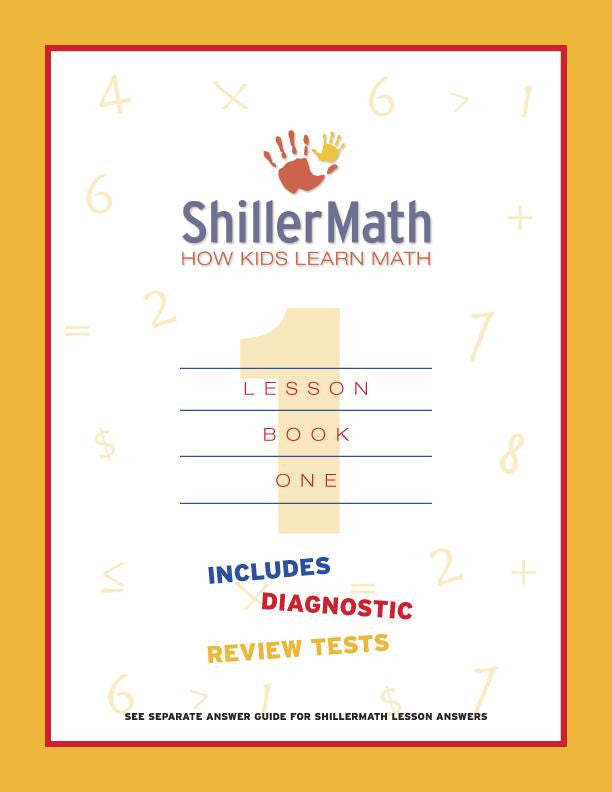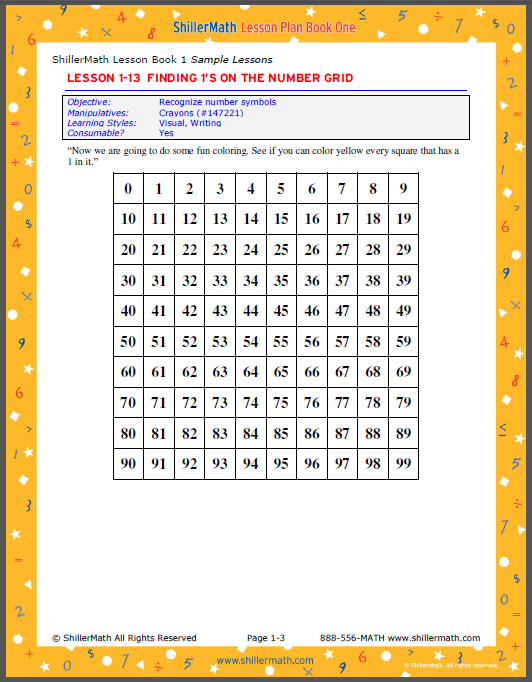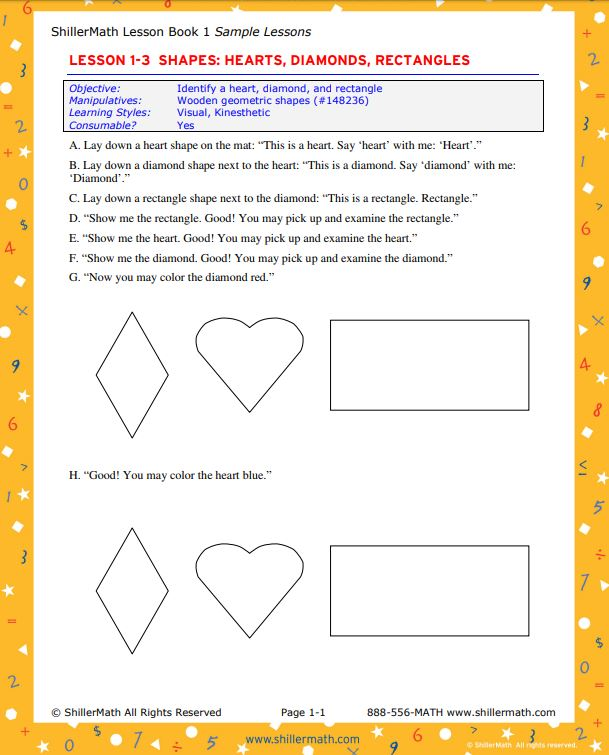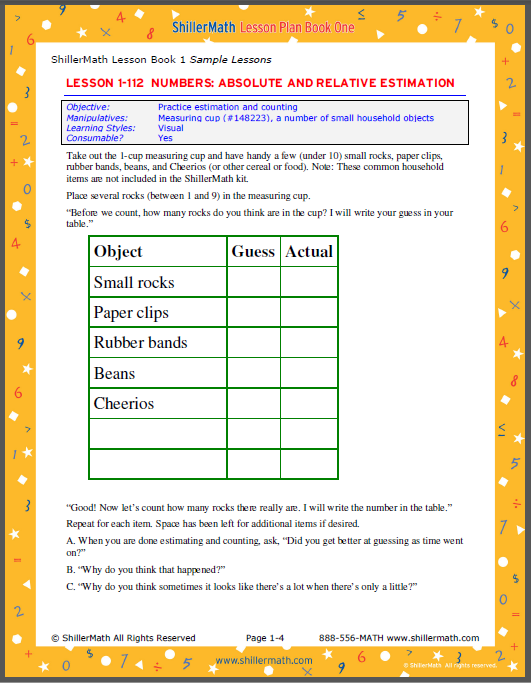## Math Lesson Book 1

### \$79.95

L01-000-0406: Hard work always leads to monumental results. Montessori-based lesson plans for 196 multi-sensory (visual, tactile, auditory, and kinesthetic) lessons and games that enable students at every level to reach their full math potential. Includes student activity pages, plus 4 diagnostic review tests and answer keys that can also be used for placement.

Math Lesson Book 1 concepts include:

• Cardinal Numbers
• Comparisons
• Counting
• Time Relative Estimation
• Direction N, S, E, W
• Equations and = sign
• Estimation
• Geometry
• Groups
• Inequalities
• Length
• Logic
• Money
• Ordinal Numbers
• Patters
• Place Value
• Polygons
• Probability
• Roman Numerals
• Rotation
• Rules
• Sets
• Signs and Symbols
• Subtraction

This book uses 14 manipulatives:

• 22” x 34” Work mat
• Songs Vol. I with 25 math songs
• Decimal material set with unit cubes, ten rods, hundred flats, and thousand cubes
• Number card set
• 14-Card operator set
• 64 Wooden geometric shapes
• Crayons
• Pair of 6-pip dice
• 1 & 2 Cup measuring cups
• 4” Foam ball
• Probability bag
• Set of 55 double-nine dominoes
• Balance
• Set of plastic US coins